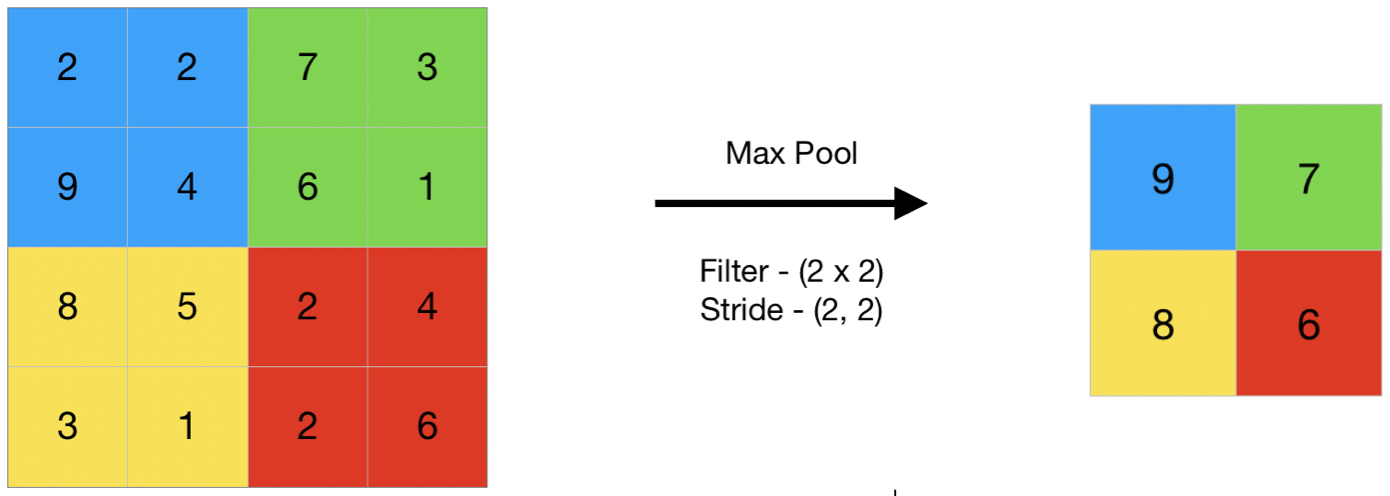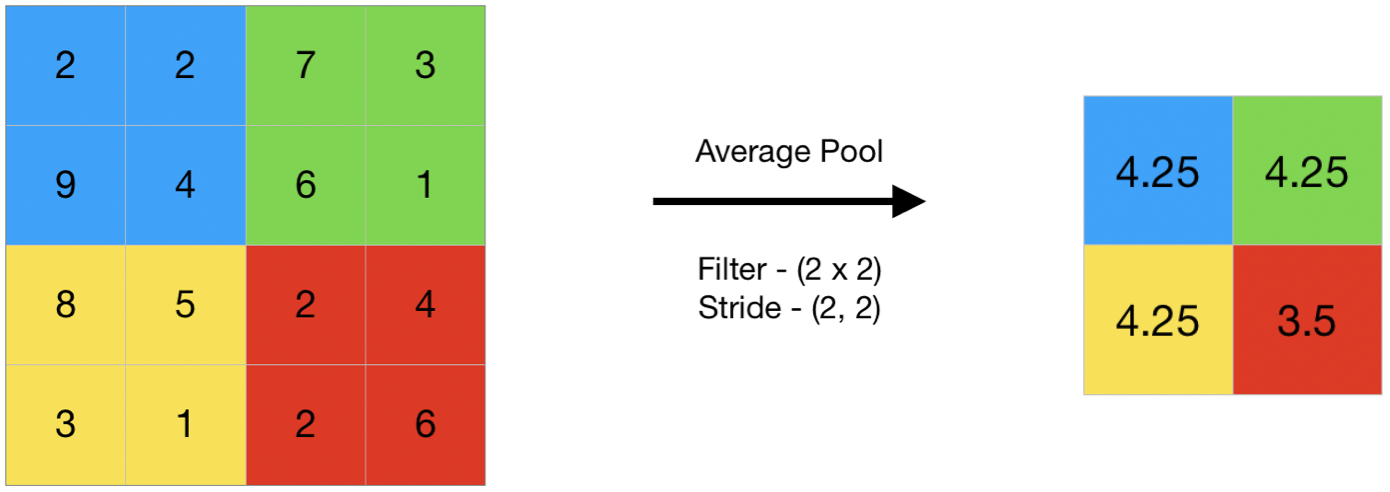# Convolutional Neural Networks• CNN are one of the most popular models used today. This neural network computational model uses a variation of multilayer perceptrons and contains one or more convolutional layers that can be either entirely connected or pooled.
• These convolutional layers create feature maps that record a region of image which is ultimately broken into rectangles and sent out for nonlinear processing.
•  Let us suppose this in the input matrix of 5×5 and a filter of matrix 3X3, for those who don’t know what a filter is a set of weights in a matrix applied on an image or a matrix to obtain the required features, please search on convolution if this is your first time!

Note: We always take the sum or average of all the values while doing a convolution

Steps Involve in CNN1. #### Edge Detection (Convolution)

• In the previous article, we saw that the early layers of a neural network detect edges from an image.Deeper layers might be able to detect the cause of the objects and even more deeper layers might detect the cause of complete objects (like a person’s face).

In this section, we will focus on how the edges can be detected from an image. Suppose we are given the below image: As you can see, there are many vertical and horizontal edges in the image. The first thing to do is to detect these edges:•  So, we take the first 3 X 3 matrix from the 7 X 7 image and multiply it with the filter. Now, the first element of the (n-k+1 x n-k+1) i.e (7-3+1 X 7-3+1) 5 X 5 output will be the sum of the element-wise product of these values, i.e. 00+00+10+10+01+00+00+10+1*0 =0. To calculate the second element of the 5 X 5 output, we will shift our filter one step towards the right and again get the sum of the element-wise product:

#### 2. Pooling

• A pooling layer is another building block of a CNN. Its function is to progressively reduce the spatial size of the representation to reduce the amount of parameters and computation in the network. Pooling layer operates on each feature map independently. The most common approach used in pooling is max pooling.
##### Types of Pooling Layers :-
###### 1. Max Pooling

Max pooling is a pooling operation that selects the maximum element from the region of the feature map covered by the filter. Thus, the output after max-pooling layer would be a feature map containing the most prominent features of the previous feature map.###### 2. Average Pooling

Average pooling computes the average of the elements present in the region of feature map covered by the filter. Thus, while max pooling gives the most prominent feature in a particular patch of the feature map, average pooling gives the average of features present in a patch.###### Problem with Simple Convolution Layers
• While applying convolutions we will not obtain the output dimensions the same as input we will lose data over borders so we append a border of zeros and recalculate the convolution covering all the input values.